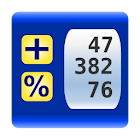gbaCalc Decimal Calculator

All Android applications categories

All Android games categories# gbaCalc Decimal Calculator

by: 20 8.8

8.8 Users
rating

## Screenshots

Description

Decimal calculator inspired by printing calculators. All operations are shown in a scrolling window like on a paper roll. Decimal fixed point with rounding, percentage and tax calculations suited for commercial use. RPN-like operation mode. Operations can be edited, saved to a file or sent by email.

www.gbizapps.com

Tags: decimal to fraction chart , decimal calculator , fraction chart , decimal chart , fraction calculation chart with decimals , code for decimal in calculator , decimal addition calculator , fraction to decimal calculator , gbacalc decimal calculator pro

from 20 reviews

"Great"

8.8